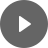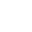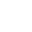16. Annuities - StudyNinja
Select Page

HSC Standard Maths Resources

Browse: 1. Home  » 16. Annuities

16. Annuities

Check out the overview section for relevant importance of this section compared to other topics in this course. In 2020 exam, two question appeared from this topic making up 4 marks (2020Q14, 2020Q37). This section covers the following parts of the syllabus:

The infographic below shows all the past exam questions from 2010 to 2020 relevant to this topic sorted by difficulty level and further broken down into sub-topics. This will form the foundation of our study as we would like you to focus first on the easy questions and quickly develop skills to get those easy marks and then challenge yourself with the harder ones.Annuity involves periodic payments over time. Questions of interest are: future value of an annuity, present value of annuity and periodic payments needed to be made to achieve a certain value in future.

The following are formulas you need to know to calculate future value (FV) and present value (PV) of a given annuity.A question can ask any part of the formula either FV or a or i or t but as long as you understand the formula well, you should be alright.

Example 1

Alan deposits \$500 at the end of each month for a year into an investment that has a return of 3% p.a. compounded monthly. What is the value of annuity after a year? What is the present value of annuity?

Instead of working out FV and PV using formula, there are times when you may be provided with a table of PV or FV for different interest rates & time periods.

For these kinds of problems, you will need to look up correct value from table and use it to answer the question.

Example 2

Following table shows the future value of \$1 invested at the end of each period at the given interest rate. What is the future value of \$350 invested at the end of each month for 3 years at 3%p.a. compounded monthly? How much needs to be invested every month so that there is \$50,000 at the end of 4 years if interest rate is 6% p.a. compounded monthly?Get access to 20 Mock Exams with over 700 exam-style questions for HSC Standard Maths.

You might find the following videos helpful related to this section:7A Annuities and Recurrence Relations (1 of 5)

by Mr Bodgers (click to view channel)

Explains how to calculate the future value of an annuity using both the recurrence relation formula and common sense methods.7A Annuities and Recurrence Relations (2 of 5)

by Mr Bodgers (click to view channel)

Completes an example where you calculate the future value of an annuity.7A Annuities and Recurrence Relations (3 of 5)

by Mr Bodgers (click to view channel)

Completes an example where the future value of an annuity is calculated using the recurrence relation formula.7A Annuities and Recurrence Relations (4 of 5)

by Mr Bodgers (click to view channel)

Completes an example where you calculate the future value of an annuity. For this example you must fill values into a table.7A Annuities and Recurrence Relations (5 of 5)

by Mr Bodgers (click to view channel)

Completes an example where you calculate the future value of an annuity. For this example repayments are made yearly into an investment account.7C Future Value Tables

by Mr Bodgers (click to view channel)

Explains how to use a table to calculate the future value of an annuity.7D Present Value Tables (1 of 2)

by Mr Bodgers (click to view channel)

Explains how to use a table to calculate the present value of an annuity. Also explains what the present value is.7D Present Value Tables (2 of 2)

by Mr Bodgers (click to view channel)

Uses a present value table to calculate a real-life scenario. The problem requires the use of a present value table.7E Annuity Problems (1 of 2)

by Mr Bodgers (click to view channel)

Explains how to interpret an annuity spreadsheet. The spreadsheet shows the growth of an investment.7E Annuity Problems (2 of 2)

by Mr Bodgers (click to view channel)

Explains how to interpret an annuity spreadsheet. The spreadsheet shows the payment of a loan.The following is the type of questions you can expect in exam:

Study notes of this section and other resources can be accessed here:

Browse: 1. Home  » 16. Annuities